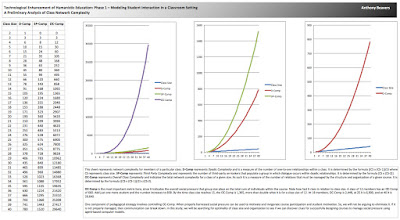## Saturday, October 31, 2015

### CLASS SIZE COMPLEXITY FOR ANY GIVEN COURSEClass Size Complexity as a Measure of the Number of Relationships in the Classroom

This diagram represents network complexity for members of a particular class. (Click on the diagram for a larger view.) D-Comp represents Dyadic Complexity and is a measure of the number of one-to-one relationships within a class. It is determined by the formula {[CS x (CS-1)]/2} where CS represents class size. 3P-Comp represents Third Party Complexity and represents the number of third-party on-lookers that populate a group in which dialogue occurs within dyadic relationships. It is determined by the formula [CS x (CS-2)]. OC-Comp represents Overall Class Complexity and indicates the total network complexity for a class of a given size. As such it is a measure of the number of relations that must be managed by the structure and organization of a given course. It is determined by the formula {[CS x (CS-1)]/2} x (CS-2).

OC-Comp is the most important metric here, since it indicates the overall social pressure that group size plays on the total sum of individuals within the course. Note how fast it rises in relation to class size. A class of 12 members has an OC-Comp of 660. Add just one more student and the number increases to 858. By the time class size reaches 15, the OC-Comp is 1,365, more than double what it is for a class size of 12. At 18 members, OC-Comp is 2,448, at 25 it is 6,900, and at 40 it is 29,640.

One component of pedagogical strategy involves controlling OC-Comp. When properly harnessed social pressure can be used to motivate and invigorate course participation and student motivation. So, we will not be arguing to eliminate it. If it is not properly managed, then communication can break down. In this study, we will be searching for optimality of class size and organization to see if we can discover clues for successfully designing courses to manage social pressure using agent-based computer models.

All factors together suggest that for a rich, discussion-based, seminar-style course, twelve students is the magic number, eleven if you count the instructor/facilitator.